Next: Single bisection mode Up: General purpose solving algorithm Previous: General purpose solving algorithm   Contents

Mathematical background

In this new algorithm we will try to improve the evaluation of the function intervals by using the Hessian of the functions. This improvement is based on a sharper analysis of the monotonicity of the functions which in turn is based on a sharper evaluation of the Jacobian matrix of the system. The element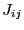of the Jacobian matrix at row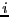and column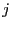is: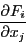Now consider the corresponding line of the Hessian matrix which is: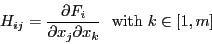The elements of this line indicate the monotonic behavior of the elements of the Jacobian matrix. If some elements in this line have a constant sign, then the elements of the Jacobian are monotonic with respect to some of the unknowns and using this monotonicity we may improve the evaluation of the element of the Jacobian matrix. This improvement has to be applied recursively: indeed as we will evaluate the Jacobian elements for boxes in which some components have now a fixed value the evaluation of the Hessian matrix for these boxes may lead to a larger number of the component of the Hessian which have a constant sign. The recursion will stop if all the component of the Hessian line have a constant sign or if the number of component with a constant sign does not increase.

Note also that not all the function must be differentiable to use this procedure: only one of them is sufficient. In that case you will have however to set special values in the gradient and hessian function (see 2.4.2.2).

We will also use the Hessian in order to try to sharpen the evaluation of. Let the box intervals be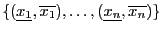. Let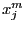be the middle point of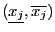and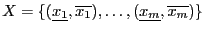be the box. Then: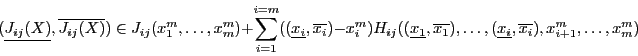(2.5)

see , pp. 52. This expression enable to get in some cases a sharper bound on.

The improvement of the evaluation of the function intervals is due to the fact that a sharper evaluation of the Jacobian matrix may lead to a larger number of Jacobian elements with constant sign than with the direct evaluation of the Jacobian matrix. To speed up the algorithm we store the Jacobian matrix of each box: this enable to avoid the evaluation of the components of the Jacobian matrix which have a constant sign when bisecting the box

Another interest of the Hessian is that it enable to use Kantorovitch theorem. This theorem (see section 3.1.2) can be applied if the number of unknowns is equal to the number of equations and enable to determine boxes in which there is an unique solution, solution which can be found using Newton method (see section 2.9) with as estimate of the solution any point within the boxes.

We will use this theorem at three possible levels:

• level 0: we want solution intervals for which the maximal width is equal or lower than a given threshold. In that case imagine that two solution intervals have been found at some point of the algorithm, this two solutions being close. We will apply Kantorovitch theorem for the center of the two solution intervals. In the most favorable case one of them will contain an unique solution while the boxes given by Kantorovitch theorem will cover the other one: consequently this input intervals will be eliminated of the solution intervals. Therefore Kantorovitch theorem will eliminate spurious solution intervals and we don't need to indicate a minimal distance between the solution intervals.
• level 1: we look for solution intervals whose width has no importance as soon as we are sure that they contain one unique solution which can be found using Newton method with as estimate of the solution any point within the solution intervals. In that case we will apply Kantorovitch theorem for each boxes which appear during the algorithm. If the theorem give a solution intervals we will store it in the solution list and update the remaining boxes of the list so that none of them contain the solution intervals. A consequence is that the width of the solution intervals cannot be determined beforehand while each solution intervals that have been determined using this method contain one unique solution which can be determined using Newton method.
• level 2: we apply Newton method for each box and if Newton converge toward a solution within the current box we store the box as solution interval. The boxes in the list are then filtered so that none of them contains the solution interval.
Furthermore we use the inflation method presented in section 3.1.6 to increase the width of the box in which we may guarantee that there is a unique solution.

As for the method using only the gradient we use the Hansen-Sengupta version of the interval Newton method to improve the boxes (see ).

SubsectionsNext: Single bisection mode Up: General purpose solving algorithm Previous: General purpose solving algorithm   Contents
Jean-Pierre Merlet 2012-12-20